# 力的计算平衡 不平衡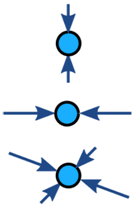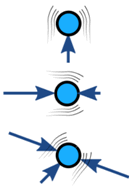无加速 加速度

### 例子：这个桥塔顶部的力是平衡的（它不在加速）：## 自由体图### 例子：高速公路上的汽车• 空气阻力（即是：空气抗拒被汽车推动），
• 滚动阻力，也叫滚动摩擦（轮胎抗拒变形）W 是汽车的重量，

R1R2 是轮胎的滚动阻力，

N1N2 是反作用力（抵消汽车的重量）。

## 计算### 例子：欲穷千里目，更上一层楼### 大山的体重

F = 80千克 × 9.81米/秒2

F = 785牛顿

### 其他的力：已知邻边，未知对边，所以我们用正切：

tan(60°) = 梁/785牛顿

cos(60°) = 785牛顿 / 支柱## 扭力（力矩）M = 785牛顿 × 3.2米 = 2512牛顿米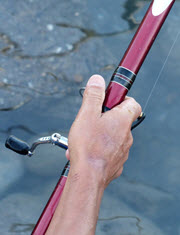## 摩擦力

### 斜面上的箱子100千克质量向下的地心引力是：

W = 100千克 × 9.81米/秒2 = 981牛顿

sin(20°) = f/981牛顿

f = sin(20°) × 981牛顿 = 336牛顿

cos(20°) = R/981牛顿

R = cos(20°) × 981牛顿 = 922牛顿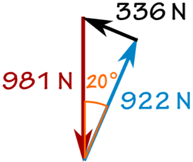## 绘画自由体图提示

• 尽量简化。通常一个方框就可以了。
• 代表力的箭头指着力施于物体的方向
• 用直的箭头来代表
• 用弯的箭头来代表力矩

## 皮皮和森森拉箱子<=>极坐标里的 矢量 a 笛卡尔坐标里的 矢量 a

将极坐标 (r,θ) 将笛卡尔坐标 (x,y) 转换为笛卡尔坐标 (x,y) 转换为极坐标 (r,θ) x = r × cos( θ ) y = r × sin( θ ) r = √ ( x2 + y2 ) θ = tan-1 ( y / x )### 例子：拉箱子

• 皮皮向 60°的方向以 200牛顿的力拉
• 森森向 45°的方向以 120牛顿的力拉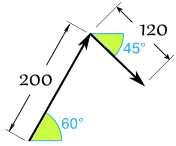• x = r × cos( θ ) = 200 × cos(60°) = 200 × 0.5 = 100
• y = r × sin( θ ) = 200 × sin(60°) = 200 × 0.8660 = 173.21

• x = r × cos( θ ) = 120 × cos(-45°) = 120 × 0.7071 = 84.85
• y = r × sin( θ ) = 120 × sin(-45°) = 120 × -0.7071 = −84.85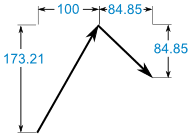(100, 173.21) + (84.85, −84.85) = (184.85, 88.36)

• r = √ ( x2 + y2 ) = √ ( 184.852 + 88.362 ) = 204.88
• θ = tan-1 ( y / x ) = tan-1 ( 88.36 / 184.85 ) = 25.5°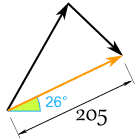"并肩作战"会好些！